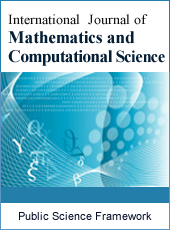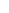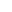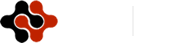International Journal of Mathematics and Computational Science
Articles Information
International Journal of Mathematics and Computational Science, Vol.5, No.1, Mar. 2019, Pub. Date: Apr. 17, 2019
Solution of Seventh Order Boundary Value Problem by Using Variational Iteration Method
 Abdulla-Al-Mamun, Department of Mathematics, Islamic University, Kushtia, Bangladesh.
 Mohammad Asaduzzaman, Department of Mathematics, Islamic University, Kushtia, Bangladesh.
In this paper, we introduce some basic idea of Variational iteration method for short (VIM) to solve the seventh order boundary value problems. It is to be mentioned that, presently, the literature on the numerical solutions of seventh order boundary value problem and associated eigen value problems is not available. By using a suitable transformation, the variational iteration method can be used to show that seventh order boundary value problems are equivalent to a system of integral equation. The VIM is used to solve effectively, easily, and accurately a large class of non-linear problems with approximations which converge rapidly to accurate solutions. For linear problems, it’s exact solution can be obtained by only one iteration step due to the fact that the Lagrange multiplier can be exactly identified. It is to be noted that the Lagrange multiplier reduces the iteration on integral operator and also minimizes the computational time. The method requires no transformation or linearization of any forms. Two numerical examples are presented to show the effectiveness and efficiency of the method. Also, we compare the result with exact solution. Finally, we investigate the error between numerical solution and exact solution and draw the graph of error function by using Mathematica.
Variational Iteration Method, Boundary Value Problem, Mathematica, Exact Solution, Approximate Solution, Numerical Solution, Lagrange Multiplier, Absolute Error
 G. Richards and P. R. R. Sarma, Reduced order models for induction motors with two rotor circuits, IEEE Transactions on Energy Conversion 9 (4) (1994), 673-678.
 J. H. He, Some asymptotic methods for strongly nonlinear equations, Int. J. Modern Phys. B 20 (10) (2006) 1141–1199.
 J. H. He, Non-perturbative methods for strongly nonlinear problems, Dissertation. de-Verlag im Internet GmbH, Berlin, 2006.
 J. H. He, Approximate analytical solution for seepage flow with fractional derivatives in porous media, Comput. Methods Appl. Mech. Engrg. 167 (1998) 57–68.
 J. H. He, Variational iteration method for autonomous ordinary differential systems, Appl. Math. Comput. 114 (2/3) (2000) 115–123.
 J. H. He, X. H. Wu, Construction of solitary solution and compacton-like solution by variational iteration method, Chaos Solitons Fractals 29 (1) (2006) 108–113.
 J. H. He, A new approach to nonlinear partial differential equations, Commun. Nonlinear Sci. Numer. Simul. 2 (4) (1997) 203–205.
 J. H. He, A variational iteration approach to nonlinear problems and its applications, Mech. Appl. 20 (1) (1998) 30–31 (in Chinese).
 J. H. He, Variational iteration method—a kind of nonlinear analytical technique: Some examples, Int. J. Nonlinear Mech. 34 (1999) 699–708.
 J. H. He, A generalized variational principle in micromorphic thermoelasticity, Mech. Res. Commun. 32 (2005) 93–98.
 J. H. He, Variational iteration method—some recent results and new interpretations, J. Comput. Appl. Math. (2006) (in press).
 E. M. Abulwafa, M. A. Abdou, A. A. Mahmoud, The solution of nonlinear coagulation problem with mass loss, Chaos Solitons Fractals 29 (2006) 313–330.
 S. Momani, Z. Odibat, Analytical approach to linear fractional partial differential equations arising in fluid mechanics, Phys. Lett. A 1 (53) (2006) 1–9.
 S. Momani, S. Abusaad, Application of He’s variational-iteration method to Helmholtz equation, Chaos Solitons Fractals 27 (5) (2005) 1119–1123.
 M. A. Abdou, A. A. Soliman, Variational iteration method for solving Burgers’ and coupled Burgers’ equation, J. Comput. Appl. Math. 181 (2005) 245–251.
 A. M. Wazwaz, A comparison between the variational iteration method and Adomian decomposition method, J. Comput. Appl. Math. (2006) (in press).
 A. M. Wazwaz, The variational iteration method for rational solutions for KdV, K(2,2), Burgers, and cubic Boussinesq equations, J. Comput. Appl. Math. (2006) (in press).
 A. M. Wazwaz, Partial Differential Equations: Methods and Applications, Balkema Publishers, The Netherlands, 2002.
 A. M. Wazwaz, Necessary conditions for the appearance of noise terms in decomposition solution series, Appl. Math. Comput. 81 (1997) 265–274.
 A. M. Wazwaz, A new technique for calculating Adomian polynomials for nonlinear polynomials, Appl. Math. Comput. 111 (1) (2000) 33–51.
 A. M. Wazwaz, A new method for solving singular initial value problems in the second order differential equations, Appl. Math. Comput. 128 (2002) 47–57.
 A. M Wazwaz, A First Course in Integral Equations, World Scientific, Singapore, 1997.
 A. M. Wazwaz, Analytical approximations and Pad´e approximants for Volterra’s population model, Appl. Math. Comput. 100 (1999) 13–25.
 G. Adomian, Solving Frontier Problems of Physics: The Decomposition Method, Kluwer, Boston, 1994.
 G. Adomian, A review of the decomposition method in applied mathematics, J. Math. Anal. Appl. 135 (1988) 501–544.
 M. Inokuti et al. General use of the Lagrange multiplier in nonlinear mathematical physics, in: S. Nemat-Nassed, ed., Variational method in the Mechanics of Solids (Pergamon Press, 1978) 156-162.
 M. Nadeem, F. Li, H. Ahmad, Nonlinear Sci. Lett. A, Vol. 9, No. 3, pp. 231-237, September 2018.
 J. H. He, Variational principle for some nonlinear partial differential equations with variable coefficients, Chaos, Solitons \& Fractals 19 (2004) 847-851.
 M. A. Abdou, A. A. Soliman, New applications of variational iteration method, Physica D 211 (1-2) (2005) 1-8.
 M. A. Abdou, A. A. Soliman, Variational iteration method for solving Burger’s and coupled Burger’s equations, J. comput. Appl. Math. 181 (2005) 245-251.
 S. Momani, S. Abuasad, Application of He’s variational iteration method to Helmholtz equation, Chaos, Solitons \& Fractals 27 (2006) 1119-1123.
 Z. M. Odibat, S. Momani, Application of variational iteration method to nonlinear differential equations of fractional order, Int. J. Nonlinear Sci. Numer. Simul. 7 (2006) 27-36.
 Pandey PK. solving third-order boundary value problems with quartic splines. Spring-plus. 2016; 5 (1): 1-10.
 S. S. Siddiqi, M. Iftikhar, Variational iteration method for the solution of seventh order boundary value problems using He’s polynomials, J. of the Association of Arab Universities for Basic and applied sciences, March 2014.
 N. Bildik, A. Konuralp, The use of variational iteration method, differential transform method and Adomian decomposition method for solving different types of nonlinear partial differential equations, Int. J. Nonlinear Sci. Numer. Simul. 7 (1) (2006) 65-70.
 M. Dehghan, M. Tatari, Identifying an unknown function in a parabolic equation with overspecified data via He’s variational iteration method, Chaos, Solitons and Fractals, Volume 36, (2008), 157-166.
 M. Dehgha, F. Shakeri, Application of He’s variational iteration method for solving the Cauchy reaction-diffusion problem, Journal of Computational and Applied Mathematics, Volume 214, (2008), 435-446.
 M. Dehgha, F. Shakeri, Numerical solution of a biological population model using He’s variational iteration method, Computers and Mathematics with Applications, 54 (2007) 1197-1209.
 M. Dehgha, F. Shakeri, Approximate solution of a differential equation arising in astrophysics using the variational iteration method, New Astronomy, 13 (2008) 53-59.
 Aslam Noor M, Mohyud-Din ST, Variational iteration technique for solving higher order boundary value problems, Computers and Mathematics with applications 189 (2007) 1929-1942.
 G. L. Liu, New research directions in the singular perturbation theory: artificial parameter approach and inverse perturbation technique, in: Proceeding of the Conference of the 7th Modern Mathematics and Mechanics, Shanghai, 1997.
 M. Inokuti, H. Sekine, and T. Mura, General use of the lagrange multiplier in nonlinear mathematical physics, Variational Method in the Mech. Of Solids, Pergamon Press, New York. (1978), 156-162.ISSN Print: 2381-7011
ISSN Online: 2381-702X
Current Issue:
Vol. 5, Issue 2, June Submit a Manuscript Join Editorial Board Join Reviewer Team
 About This Journal All Issues Open Access Indexing Payment Information Author Guidelines Review Process Publication Ethics Editorial Board Peer Reviewers600 ATLANTIC AVE, BOSTON,
MA 02210, USA+001-6179630233
JournalsJournal ListsAuthor GuidelinesPayment InformationSubmit a ManuscriptJoin as Editorial MembersJoin as ReviewersPrivacy NoticeTerms of ConditionsHelp Center